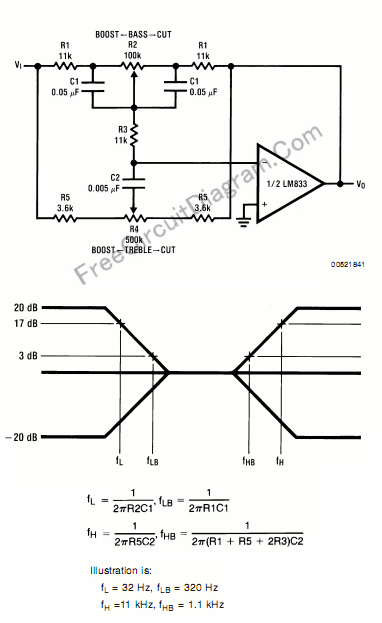# Baxandall Tone Control Using LM833: The Circuit’s Schematic Diagram and The FormulaThis circuit is similar to our previous tone control circuit, a bass-treble tone control circuit, but here we present the formula for design your tone control’s custom frequency response. Here is the schematic diagram and formula of the tone control circuit:Almost all op-amp type can be used, as long as they have high input impedance, much higher than the resistors selection. The resistor should be much lower value than the capacitor’s leakage resistance equivalence value. Finally, using the formula shown in the above figure, we can design custom frequency response for our own Baxandall tone control circuit. [Schematic diagram source: National Semiconductor Application Notes]